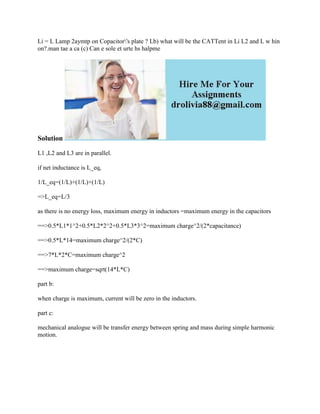Se está descargando tu SlideShare. ×

# Li - L Lamp 2aymtp on Copacitor-'s plate - Lb) what will be the CATTen.docx

Anuncio
Anuncio
Anuncio
Anuncio
Anuncio
Anuncio
Anuncio
Anuncio
Anuncio
Anuncio
AnuncioCargando en…3
×

1 de 2 Anuncio

# Li - L Lamp 2aymtp on Copacitor-'s plate - Lb) what will be the CATTen.docx

Li = L Lamp 2aymtp on Copacitor\'s plate ? Lb) what will be the CATTent in Li L2 and L w hin on?.man tae a ca (c) Can e sole et urte hs halpme
Solution
L1 ,L2 and L3 are in parallel.
if net inductance is L_eq,
1/L_eq=(1/L)+(1/L)+(1/L)
=>L_eq=L/3
as there is no energy loss, maximum energy in inductors =maximum energy in the capacitors
==>0.5*L1*1^2+0.5*L2*2^2+0.5*L3*3^2=maximum charge^2/(2*capacitance)
==>0.5*L*14=maximum charge^2/(2*C)
==>7*L*2*C=maximum charge^2
==>maximum charge=sqrt(14*L*C)
part b:
when charge is maximum, current will be zero in the inductors.
part c:
mechanical analogue will be transfer energy between spring and mass during simple harmonic motion.
.

Li = L Lamp 2aymtp on Copacitor\'s plate ? Lb) what will be the CATTent in Li L2 and L w hin on?.man tae a ca (c) Can e sole et urte hs halpme
Solution
L1 ,L2 and L3 are in parallel.
if net inductance is L_eq,
1/L_eq=(1/L)+(1/L)+(1/L)
=>L_eq=L/3
as there is no energy loss, maximum energy in inductors =maximum energy in the capacitors
==>0.5*L1*1^2+0.5*L2*2^2+0.5*L3*3^2=maximum charge^2/(2*capacitance)
==>0.5*L*14=maximum charge^2/(2*C)
==>7*L*2*C=maximum charge^2
==>maximum charge=sqrt(14*L*C)
part b:
when charge is maximum, current will be zero in the inductors.
part c:
mechanical analogue will be transfer energy between spring and mass during simple harmonic motion.
.

Anuncio
Anuncio

### Li - L Lamp 2aymtp on Copacitor-'s plate - Lb) what will be the CATTen.docx

1. 1. Li = L Lamp 2aymtp on Copacitor's plate ? Lb) what will be the CATTent in Li L2 and L w hin on?.man tae a ca (c) Can e sole et urte hs halpme Solution L1 ,L2 and L3 are in parallel. if net inductance is L_eq, 1/L_eq=(1/L)+(1/L)+(1/L) =>L_eq=L/3 as there is no energy loss, maximum energy in inductors =maximum energy in the capacitors ==>0.5*L1*1^2+0.5*L2*2^2+0.5*L3*3^2=maximum charge^2/(2*capacitance) ==>0.5*L*14=maximum charge^2/(2*C) ==>7*L*2*C=maximum charge^2 ==>maximum charge=sqrt(14*L*C) part b: when charge is maximum, current will be zero in the inductors. part c: mechanical analogue will be transfer energy between spring and mass during simple harmonic motion.